ELibM Book Reviews    For fastest access: Choose your nearest mirror site!
This book review is an expanded version of the ZMATH review of
Feldman, Joel; Knörrer, Horst; Trubowitz, Eugene:
Fermionic functional integrals and the renormalization group.
CRM Monograph Series 16. Providence, RI: American Mathematical Society (2002).

This text is also available as: DVI [12 kilobytes] PostScript [104 kilobytes] PDF [56 kilobytes]

# Fermionic Functional Integrals and the Renormalization Group

### CRM Monograph Series 16. Providence, RI: American Mathematical Society. 114 p. \$ 35.00 (2002) [ISBN 0-8218-2878-9; ISSN 1065-8599]

Book reviewed by Manfred Salmhofer

This book is based on the Aisenstadt lectures of Joel Feldman at the CRM in Montréal. It provides an exposition of techniques for the construction of interacting fermionic quantum field theories. The mathematical construction of a Fermi liquid by Feldman, Knörrer and Trubowitz has since then appeared in a series of 11 papers . The book reviewed here can be considered as an introduction to these papers.

# The Setting

The functional integral approach to quantum field theory has become a standard tool of theoretical physics. In relativistic theories, it allows for a manifestly covariant formulation of the theory, and it has given rise to many important insights and developments, e.g. in gauge theories.

Functional integrals are notorious for being mathematically undefined and much effort has been made to change this situation. In quantum mechanics, the transition to imaginary time, i.e. replacing the time evolution operator by the heat kernel, leads to the Feynman-Kac formula which involves mathematically well-defined integrals with respect to Wiener measure (see, e.g. ). Similarly, the Wick rotation to imaginary time in quantum field theory gives a connection to statistical mechanics that generalizes the Feynman-Kac formula and allows for mathematically rigorous constructions of such theories. A Wightman theory in Minkowski space can be reconstructed provided the Osterwalder-Schrader axioms are satisfied by the Schwinger functions of the Euclidian theory . Proving that the Schwinger functions associated to certain Lagrangians exist and satisfy these axioms is the program of constructive quantum field theory. Several books describe the progress in that direction [4,5]. These constructions are in general rather complicated because almost all models involve bosonic fields which require a combination of cluster expansions and large-field bounds in addition to renormalization.

In nonrelativistic theories corresponding to the quantum statistical mechanics of many-body systems, functional integrals have become a natural and very useful tool which by now is completely standard in theoretical solid-state physics. Theories of electrons with a short-range two-body interaction can be formulated as purely fermionic theories, which allow for a much simpler treatment that avoids cluster expansions. The book describes such techniques.

Physically interesting functions in second-quantized fermion systems can be represented as fermionic functional integrals over suitable Grassmann algebras. For instance, the free energy density of a system of fermions with Hamiltonian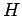in a finite volume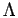at inverse temperature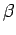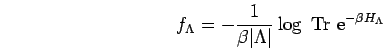(1)

can formally be written as a Grassmann Gaussian integral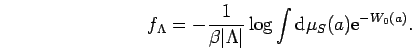(2)

The covariance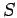, an antisymmetric operator, and the interaction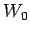, an even element of the Grassmann algebra, are determined by the Hamiltonian of the model. The hard mathematical problem is to prove that the right hand side of (2), as well as suitable generalizations thereof, exists and has a limit as the volume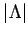tends to infinity, in the physically interesting region of low temperatures.

The methods of constructive field theory, in particular the mathematical renormalization group (RG) method are well suited to treating this problem. Moreover, they give direct access to physical properties, namely equilibrium properties of such systems, without invoking Osterwalder-Schrader reconstruction theorems.

The constructive field theory approach to many-body systems was pioneered by Feldman and Trubowitz  and independently by Benfatto and Gallavotti , and has since then been used by these authors and others to prove a number of mathematical theorems about solid state systems which were not accessible to other methods. Variations of the same RG technique are being increasingly used as calculational tools in solid state theory [8,9].

# Overview of the book

The book is divided into two parts. Part 1 contains an introduction to Grassmann algebra and fermionic functional integrals of the type appearing on the right hand side of (2). The renormalization group transformation is introduced to deal with singular covariances which are characteristic of physically interesting problems. In Part 2, some aspects of the construction of fermionic models by the methods of Part 1 are treated.

## Part 1

Finite-dimensional Grassmann algebras and superalgebras are introduced in Section 1.1. Sections 1.2 and 1.3 contain standard material about differentiation and integration as linear maps on Grassmann algebras. Grassmann Gaussian Integrals are introduced and in Section 1.4 their connection to Pfaffians and determinants is reviewed. Proposition 1.21 contains the addition principle for Gaussian integration, which is the basis for the integration step in the RG transformation. In Section 1.5, the above-mentioned relation of Grassmann integrals and fermionic quantum field theories in second quantized Hamiltonian form is reviewed, and formulas generalizing (2) to a complete set of observables, the time-ordered functions, from which all observables can be reconstructed, are stated without proof. Because all interesting covariances are singular, the construction requires taking a limit. The singular covarianceis written as an infinite sum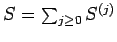of regular ones. The addition principle allows to do the Gaussian integral successively, integrating with covariance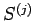in step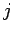. This leads in a natural way to the definition of the RG map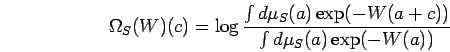(3)

Basic properties of the map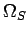are discussed in Section 1.6. The antisymmetry of the Grassmann generators implies almost trivially that normalized integrals are rational functions in the interaction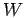(this property does not hold in bosonic theories, where Grassmann algebras are replaced by algebras with commuting generators). The Gaussian integral with respect tois thus calculated by an infinite iteration of RG maps, which generate a sequence of effective interactions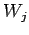by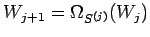. The problem is thus to prove that this sequence converges in a suitable Banach space of interactions.

Section 1.7 contains some facts about Wick ordering, an important technical ingredient. Wick ordering with respect to a Gaussian measure is simply introducing orthogonal polynomials for this measure, and the fermionic version is a straightforward variant of it. Wick ordering is not fundamental, but it simplifies some calculations and bounds, in particular the identification of overlapping loops'' , which are not discussed in this monograph but are crucial for the construction of the Fermi liquid in . Wick ordering is also an important ingredient in the ring expansion developed in Part 2 of the book.

The treatment in all of Part 1 is for finite-dimensional algebras, while the object appearing on the right hand side of (2) is a formal, infinite-dimensional Grassmann integral. The calculus on finite-dimensional Grassmann algebras developed in part 1 extends in a natural way to infinite-dimensional ones if the covariance of the Gaussian integral is sufficiently regular. Ways to do this are discussed briefly in Appendix A. This extension does not play a central role in the construction because the integral with a singular covariance on the RHS of (2) has to be approached by taking a limit anyway, and because in most applications, one can construct it as a limit of finite-dimensional Grassmann integrals, to which the methods of Part 1 apply directly. For instance, for a lattice system, whereis a finite set of cardinality, the dimension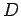of the Grassmann algebra diverges as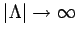. Thus one needs to prove bounds that are uniform in.

For finite, the covarianceis just an antisymmetric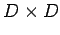matrix. Section 1.8 contains important bounds for the analytical treatment of the Grassmann integrals, the classic Gram estimate: if an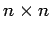matrix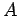has entries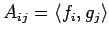where for all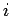and,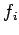and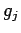are vectors in some Hilbert space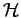, then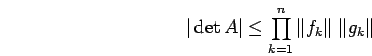(4)

If the covariancehas matrix elements of this form, the Gram estimate implies analyticity of the RG map infor a general class of interactions. The covariances of fermionic field theories are of this form since they are given as Fourier transforms. For the covariances,fixed, the norms of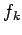and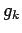are uniformly bounded in. In the many-fermion systems this is true even uniformly in.

## Part 2

The Gram estimate alone does, however, not imply analyticity of the renormalization group map inuniformly in. For this, a sufficiently fast decay of the covariance is also needed, i.e.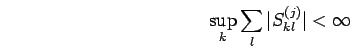(5)

In the many-fermion systems and for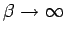, this decay is true only for the covariances, not foritself. That is,is singular because of its slow decay.

To prove uniform analyticity, one also needs to exhibit certain connectedness properties of the logarithm of ratios like the one in (3). The connectedness property is stated most easily in terms of a Feynman graph expansion: only connected graphs contribute. It is this property that ensures that, e.g. the free energy density defined in (1) indeed has a limit as. The full expansion into Feynman graphs cannot be used because graph-by-graph estimates lead to zero radius of analyticity. Connectedness can be made explicit in a way that allows for convergent bounds in several ways. In their work, the authors choose the ring expansion''. In Section 2.2, they rewrite the Schwinger functional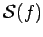(Definition 2.1), from which one can obtain the RG map, in terms of an operator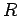acting on the Grassmann algebra as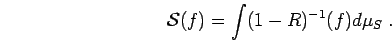(6)is sometimes called the ring operator because, in a representation of the contributions to the Gaussian integral by Feynman graphs, its application can be pictorialized as adding layers to Feynman graphs, starting from a distinguished vertex . This graphical representation is not needed in the proofs, but it provides some useful intuition.

The crucial hypotheses for the proof of analyticity, as formulated in Theorem 2.6 and Corollary 2.7, are in Definition 2.5. Hypothesis (HG) is a restatement of the Gram bound, and (HS) is the summability of the covariance given by (5).

In Section 2.4, some applications are given. The first example is the two-dimensional Gross-Neveu model. The covariance is the inverse of a Dirac operator. The interaction is a local four-fermion interaction. The limit to be taken is an ultraviolet limit, i.e. the singularity is in the short-distance behaviour of the covariance. This model is interesting because it is perturbatively just renormalizable, with power counting very similar to scalartheory in 4 dimensions, but a construction of this model has been possible [11,12] because it is UV asymptotically free.

After this, the many-fermion system in two spatial dimensions is treated. The big difference to the previous case is that the singularity of the many-fermion covariance in Fourier space is not pointlike but on a submanifold of codimension one, the Fermi surface (in two dimensions a curve). A treatment along the lines sufficient for point singularities is presented as a warmup. It gives bounds that are too weak to control the-behaviour in a good way. After that, the sectorization'', an angular decomposition of the Fermi curve, is introduced and a basic convergence theorem (Theorem 2.15 MB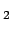) is proven. The introduction of sectors allows to use geometrical restrictions posed by the shape of the Fermi curve, to improve the bounds such that physically interesting models can be constructed. The sectorization, first introduced in , is a central technical tool in the study of these systems in two dimensions. The bounds for the Gram constant and the decay constant of the covariance are given in Appendix C.

In all these applications, the bounds given in the book are restricted to those interaction terms that decrease under the iteration of the RG map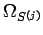(the irrelevant terms in RG language). That is, a straightforward iteration inof these bounds is not possible because of the hypothesis that the part of degree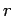in the Grasssmann generators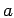,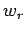, vanishes for small values of(e.g.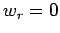for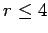in Theorem 2.15 MB). Even if this holds for, it does not hold for, so one cannot iterate. In fact, thewith smallare those that tend to grow under iteration of the RG map, and they have to be controlled by a careful choice of counterterms and initial conditions, as well as a much more detailed analysis of the RG map and its iteration. This is a hard task which takes up most of  and other constructions of these models, and not treated in the book. The bounds in the book do imply that one can focus on the flow of thefor small. These functions are also the ones that one restricts to in practical applications to physics.

# Concluding remarks

It is clearly not the intent of the authors to provide a very detailed discussion of the background of the problem and its motivation. Their aim is to expose their method as briefly as possible. Conciseness is indeed one of the great virtues of the book: parts 1 and 2 are essentially 30 pages each, but they are selfcontained, and in particular part 2 contains important partial results of the construction in . The text is written very clearly and carefully. Moreover, many problems are included, and complete solutions are provided in Appendix D.

Unfortunately, there is almost no discussion of how the results derived in the book fit into the general strategy of . There is also no mention, not even a citation of other works on the same class of problems using essentially the same RG strategy but slightly different techniques or focusing on slightly different situations. The ring expansion is not the only way to organize expansions such that analyticity statements can be proven. The same is also possible using tree expansions à la Brydges-Battle-Federbush [14,15] or bounds obtained from the Brydges-Kennedy formulas [16,17]. The organization of the expansions using Laplacians in the field variables done in some of these works provides an alternative to the ring expansion technique. An introduction to the algebraic aspects, some background, as well as a detailed proof of the relation to the second-quantized Hamiltonian formulation, and a list of relevant references, is provided, e.g., in . Another recent reference about the RG is .

To summarize, this is an excellent technical introduction for graduate students and researchers new to the field who want to start reading the series of papers . To get a motivation and a larger perspective of the study of these problems, or to learn about alternative techniques, readers need to consult other references.

## Bibliography

1
J. Feldman, H. Knörrer, E. Trubowitz, Rev. Math. Phys. 15 (2003) 949-994, 995-1038, 1039-1120, 1121-1169, Comm. Math. Phys., in press

2
B. Simon, Functional Integration and Quantum Physics, Academic Press, New York, 1979

3
K. Osterwalder, R. Schrader, Comm. Math. Phys. 31, 83; 42, 281

4
J. Glimm, A. Jaffe, Quantum Physics: A functional integral point of view. Springer, Heidelberg, 1987

5
V. Rivasseau, From Perturbative to Constructive Renormalization, Princeton University Press, 1991

6
J. Feldman, E. Trubowitz, Helv. Phys. Acta 63 (1990) 157, 64 (1991) 213

7
G. Benfatto, G. Gallavotti, J. Stat. Phys. 59 (1990) 541

8
C. Halboth, W. Metzner, Phys. Rev. B 61 (2000) 7364; Phys. Rev. Lett. 85 (2000) 5162

9
C. Honerkamp, M. Salmhofer, N. Furukawa, T.M. Rice, Phys. Rev. B 63, 035109 (2001)

10
J. Feldman, M. Salmhofer, E. Trubowitz, J. Stat. Phys. 84 (1996) 1209-1336

11
K. Gawedzki, A. Kupiainen, Comm. Math. Phys. 102 (1985) 1-30

12
J. Feldman, J. Magnen, V. Rivasseau, R. Sénéor, Phys. Rev. Lett. 54 (1985) 1479-1481 Comm. Math. Phys.

13
J. Feldman, J. Magnen, V. Rivasseau, E. Trubowitz, Helv. Phys. Acta 65 (1992) 679

14
A. Lesniewski, Comm. Math. Phys. 108 (1987) 437-467

15
M. Salmhofer, C. Wieczerkowski, J. Stat. Phys. 99 (2000) 557-586

16
A. Abdesselam, V. Rivasseau, Lett. Math. Phys. 41 (1998) 77-88

17
M. Disertori, V. Rivasseau, Comm. Math. Phys. 215, 251,291 (2000)

18
M. Salmhofer, Renormalization: An Introduction, Springer Texts and Monographs in Physics, Springer, Heidelberg, 1998

19
G. Benfatto, G. Gallavotti, Renormalization Group, Princeton University Press, 1995

Manfred Salmhofer
Institut für Theoretische Physik
Universität Leipzig
Augustusplatz 10
04109 Leipzig

Email: salmhofer@itp.uni-leipzig.de

Publication date: 28 Apr 2004
© 2004 ELibM and FIZ Karlsruhe / Zentralblatt MATH. All rights reserved.